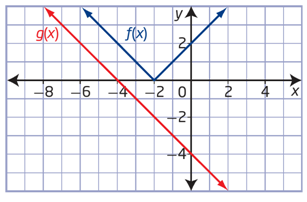# Composite functions##### Introduction
###### Lessons
1. What is a "Composite Function"?
2. Quick review on basic operations with functions.
3. Composition of Functions: putting one function inside another function!
##### Examples
###### Lessons
1. Introduction to Composite Functions
If $f(x)= 4x-5$
$g(x)=8x^2+6$
Determine
1. $(f\circ g)(x)$
2. $(g\circ f)(x)$
3. $(f\circ f)(x)$
4. $(g\circ g)(x)$
5. $(f\circ g)(2)$
evaluate in two different ways
2. Evaluate a Composite Function Graphically
Use the graphs of $f(x)$ and $g(x)$ to evaluate the following:1. $f(g(-4))$
2. $f(g(0))$
3. $g(f(-2))$
4. $g(f(-3))$
3. Determine the Composition of Three Functions
Use the functions $f(x)=3x,$
$g(x)=x-7$
and $h(x)=x^2$
to determine each of the following:
1. $(f\circ g\circ h)(x)$
2. $g(f(h(x)))$
3. $f(h(g(x)))$
4. $(h\circ g\circ f)(x)$
4. Determine the Original Functions From a Composite Function
If $h(x)=(f\circ g)(x)$
determine $f(x)$
and $g(x)$
1. $h(x)=(7x-5)^3-4(7x-5)+1$
2. $h(x)=\sqrt{4x^3-9}$, give two possible sets of solutions
5. Composite Functions with Restrictions
Consider $f(x)=\sqrt{x-13}$
and $g(x)=x^2+4$, for each of the function below:
1. Determine:
i)
$(f\circ g)(x)$
ii)
$(g\circ f)(x)$
2. State the domain and range of:
i)
$f(x)$
ii)
$g(x)$
iii) $(f\circ g)(x)$
iv)
$(g\circ f)(x)$
3. Sketch the graph of:
i)
$f(x)$
ii)
$g(x)$
iii) $(f\circ g)(x)$
iv)
$(g\circ f)(x)$
6. Composite Functions with Restrictions
Consider $f(x)=\sqrt{x-13}$
and $g(x)=x^2+4$, for each of the function below:
i) state the domain and range
ii) sketch the graph.
1. $f(x)$
2. $g(x)$
3. $(f\circ g)(x)$
###### Free to Join!
StudyPug is a learning help platform covering math and science from grade 4 all the way to second year university. Our video tutorials, unlimited practice problems, and step-by-step explanations provide you or your child with all the help you need to master concepts. On top of that, it's fun - with achievements, customizable avatars, and awards to keep you motivated.
• #### Easily See Your ProgressWe track the progress you've made on a topic so you know what you've done. From the course view you can easily see what topics have what and the progress you've made on them. Fill the rings to completely master that section or mouse over the icon to see more details.
• #### Make Use of Our Learning Aids###### Practice Accuracy

See how well your practice sessions are going over time.

Stay on track with our daily recommendations.

• #### Earn Achievements as You LearnMake the most of your time as you use StudyPug to help you achieve your goals. Earn fun little badges the more you watch, practice, and use our service.
• #### Create and Customize Your AvatarPlay with our fun little avatar builder to create and customize your own avatar on StudyPug. Choose your face, eye colour, hair colour and style, and background. Unlock more options the more you use StudyPug.